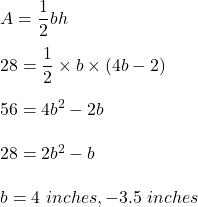Question

a triangle with area 28 square inches has a height that is twi less than four times the base. find the base and the height if the tringle?

1.b = 4 inches and h = 14 inches

Step-by-step explanation:

Given that,

The area of a triangle, A = 28 sq inches

Height is twice less than 4 times the base.

H = 4b-2, b is base

We know that,

The area of a triangle is given by :Base = 4 inches

Height = 4(4)-2

= 14 inches

Hence, this is the required solution.